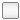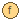## FCSys.Utilities

General supporting functions

### Information

Extends from Modelica.Icons.UtilitiesPackage (Icon for utility packages).

### Package Content

NameDescriptionChemistry Functions to support chemistryCoordinates Functions to handle Cartesian coordinatesMeans Package of mathematical mean functionsPolynomial Polynomial functionsTime Functions to check translation timearrayBooleanEqual Check if two `Boolean` vectors are equalarrayIntegerEqual Check if two `Integer` vectors are equalarrayRealEqual Check if two `Real` vectors are nearly equalarrayStringEqual Check if two vectors of strings are equalassertEval Assert function that forces Dymola to parse the messageDelta Return the second entry of a vector minus the first (Δ)inSign Return the mathematical sign for the direction into a side or boundarymod1 Modulo operator for 1-based indexing (compatible with Modelica)plot6 Create six plotsround Round a `Real` variable to the nearest integerselectBooleans Reduce a `Boolean` vector by selecting indicesselectIntegers Reduce an `Integer` vector by selecting indicesSigma Return the sum of the first and second entries of a vector (Σ)

##FCSys.Utilities.arrayBooleanEqual

Check if two `Boolean` vectors are equal

### Information

Examples:
`arrayIntegerEqual({1,2}, {1,2})` returns `true`, but `arrayIntegerEqual({1,2}, {1,3})` and `arrayIntegerEqual({1,2}, {1,2,3})` each return false. Extends from Modelica.Icons.Function (Icon for functions).

### Inputs

TypeNameDefaultDescription
Booleanu1[:] First Boolean vector
Booleanu2[:] Second Boolean vector

### Outputs

TypeNameDescription
Booleanequal`true`, if all of the entries are equal

### Modelica definition

```function arrayBooleanEqual
"Check if two `Boolean` vectors are equal"
extends Modelica.Icons.Function;

input Boolean u1[:] "First Boolean vector";
input Boolean u2[:] "Second Boolean vector";
output Boolean equal
"`true`, if all of the entries are equal";

algorithm
if size(u1, 1) <> size(u2, 1) then
equal := false;
return;
end if;
for i in 1:size(u1, 1) loop
if u1[i] <> u2[i] then
equal := false;
return;
end if;
end for;
equal := true;
return;
end arrayBooleanEqual;
```

##FCSys.Utilities.arrayIntegerEqual

Check if two `Integer` vectors are equal

### Information

Examples:
`arrayIntegerEqual({1,2}, {1,2})` returns `true`, but `arrayIntegerEqual({1,2}, {1,3})` and `arrayIntegerEqual({1,2}, {1,2,3})` each return false. Extends from Modelica.Icons.Function (Icon for functions).

### Inputs

TypeNameDefaultDescription
Integeru1[:] First integer vector
Integeru2[:] Second integer vector

### Outputs

TypeNameDescription
Booleanequal`true`, if all of the entries are equal

### Modelica definition

```function arrayIntegerEqual
"Check if two `Integer` vectors are equal"
extends Modelica.Icons.Function;

input Integer u1[:] "First integer vector";
input Integer u2[:] "Second integer vector";
output Boolean equal
"`true`, if all of the entries are equal";

algorithm
if size(u1, 1) <> size(u2, 1) then
equal := false;
return;
end if;
for i in 1:size(u1, 1) loop
if u1[i] <> u2[i] then
equal := false;
return;
end if;
end for;
equal := true;
return;
end arrayIntegerEqual;
```

##FCSys.Utilities.arrayRealEqual

Check if two `Real` vectors are nearly equal

### Information

Examples:
`arrayRealEqual({1,2}, {1,2})` returns `true`, but `arrayRealEqual({1,2}, {1,2.001})` and `arrayRealEqual({1,2}, {1,2,3})` each return false. Extends from Modelica.Icons.Function (Icon for functions).

### Inputs

TypeNameDefaultDescription
Realu1[:] First real vector
Realu2[:] Second real vector
Realepsilon1e-7Error tolerance

### Outputs

TypeNameDescription
Booleanequal`true`, if all of the entries are equal

### Modelica definition

```function arrayRealEqual
"Check if two `Real` vectors are nearly equal"
extends Modelica.Icons.Function;

input Real u1[:] "First real vector";
input Real u2[:] "Second real vector";
input Real epsilon=1e-7 "Error tolerance";
output Boolean equal
"`true`, if all of the entries are equal";

algorithm
if size(u1, 1) <> size(u2, 1) then
equal := false;
return;
end if;
for i in 1:size(u1, 1) loop
if abs(u1[i] - u2[i]) > epsilon then
equal := false;
return;
end if;
end for;
equal := true;
return;
end arrayRealEqual;
```

##FCSys.Utilities.arrayStringEqual

Check if two vectors of strings are equal

### Information

Examples:
`arrayStringEqual({"a","bc"}, {"a","bc"})` returns `true`, but `arrayStringEqual({"a","bc"}, {"a","b"})` and `arrayStringEqual({"a","b"}, {"a","b","c"})` each return false. Extends from Modelica.Icons.Function (Icon for functions).

### Inputs

TypeNameDefaultDescription
Stringu1[:] First string vector
Stringu2[:] Second string vector

### Outputs

TypeNameDescription
Booleanequal`true`, if all of the entries are equal

### Modelica definition

```function arrayStringEqual "Check if two vectors of strings are equal"
extends Modelica.Icons.Function;

input String u1[:] "First string vector";
input String u2[:] "Second string vector";
output Boolean equal
"`true`, if all of the entries are equal";

algorithm
if size(u1, 1) <> size(u2, 1) then
equal := false;
return;
end if;
for i in 1:size(u1, 1) loop
if u1[i] <> u2[i] then
equal := false;
return;
end if;
end for;
equal := true;
return;
end arrayStringEqual;
```

##FCSys.Utilities.assertEval

Assert function that forces Dymola to parse the message

### Information

When an assert statement is false in the initial equation section of a model, Dymola 2014 gives the following error during translation:

`"Error: The conditions of the following assert statements are always false:"`

without parsing the message given to the assert function. This pass-through function causes the statement to be evaluated during initialization, at which point the message is evaluated.

Extends from Modelica.Icons.Function (Icon for functions).

### Inputs

TypeNameDefaultDescription
Booleancondition
Stringmessage

### Modelica definition

```function assertEval
"Assert function that forces Dymola to parse the message"
extends Modelica.Icons.Function;
input Boolean condition;
input String message;

algorithm
assert(condition, "\n" + message + "\n");
end assertEval;
```

##FCSys.Utilities.Delta

Return the second entry of a vector minus the first (Δ)

### Information

The translator should automatically vectorize (or "matricize") this function. For example, `Delta([1,2;3,4])` returns `{1,1}`.

Extends from Modelica.Icons.Function (Icon for functions).

### Inputs

TypeNameDefaultDescription
Realu Vector of size two

### Outputs

TypeNameDescription
RealDeltaSecond entry minus the first entry

### Modelica definition

```function Delta
"Return the second entry of a vector minus the first (Δ)"
extends Modelica.Icons.Function;
input Real u "Vector of size two";
output Real Delta "Second entry minus the first entry";

algorithm
Delta := u - u;
end Delta;
```

##FCSys.Utilities.inSign

Return the mathematical sign for the direction into a side or boundary

### Information

Examples:
`inSign(FCSys.BaseClasses.Side.n)` returns 1 and `inSign(FCSys.BaseClasses.Side.p)` returns -1. Extends from Modelica.Icons.Function (Icon for functions).

### Inputs

TypeNameDefaultDescription
Sideside Side

### Outputs

TypeNameDescription

### Modelica definition

```function inSign
"Return the mathematical sign for the direction into a side or boundary"
extends Modelica.Icons.Function;
input Side side "Side";

algorithm
sign := 3 - 2*side;
end inSign;
```

##FCSys.Utilities.mod1

Modulo operator for 1-based indexing (compatible with Modelica)

### Information

Examples:
`mod1(4,3)` returns 1. `mod1(3,3)` returns 3, but `mod(3,3)` returns 0 (where `mod` is the built-in modulo operator). Extends from Modelica.Icons.Function (Icon for functions).

### Inputs

TypeNameDefaultDescription
Integernum Dividend
Integerden Divisor

### Outputs

TypeNameDescription
IntegerindexRemainder with 1-based indexing

### Modelica definition

```function mod1
"Modulo operator for 1-based indexing (compatible with Modelica)"
extends Modelica.Icons.Function;
input Integer num "Dividend";
input Integer den "Divisor";
output Integer index "Remainder with 1-based indexing";

algorithm
index := mod(num - 1, den) + 1;
end mod1;
```

##FCSys.Utilities.plot6

Create six plots

### Information

This function calls the `createPlot()` function in Dymola to create a tiled array of six plots. It may not work with other tools.

Extends from Modelica.Icons.Function (Icon for functions).

### Inputs

TypeNameDefaultDescription
Stringy1[:]fill("", 0)Names of the signals for the 1st plot
Stringy2[:]fill("", 0)Names of the signals for the 2nd plot
Stringy3[:]fill("", 0)Names of the signals for the 3rd plot
Stringy4[:]fill("", 0)Names of the signals for the 4th plot
Stringy5[:]fill("", 0)Names of the signals for the 5th plot
Stringy6[:]fill("", 0)Names of the signals for the 6th plot

### Modelica definition

```function plot6 "Create six plots"
extends Modelica.Icons.Function;
input String y1[:]=fill("", 0)
"Names of the signals for the 1st plot";
input String y2[:]=fill("", 0)
"Names of the signals for the 2nd plot";
input String y3[:]=fill("", 0)
"Names of the signals for the 3rd plot";
input String y4[:]=fill("", 0)
"Names of the signals for the 4th plot";
input String y5[:]=fill("", 0)
"Names of the signals for the 5th plot";
input String y6[:]=fill("", 0)
"Names of the signals for the 6th plot";

algorithm
createPlot(
id=1,
position={0,0,440,650},
y=y1,
erase=false,
grid=true,
online=true,
legendLocation=5,
legendHorizontal=false,
leftTitleType=1,
bottomTitleType=1);
createPlot(
id=1,
position={0,0,440,325},
y=y2,
erase=false,
grid=true,
online=true,
legendLocation=5,
legendHorizontal=false,
subPlot=2,
leftTitleType=1,
bottomTitleType=1);
createPlot(
id=2,
position={450,0,440,650},
y=y3,
erase=false,
grid=true,
legendLocation=5,
legendHorizontal=false,
online=true,
leftTitleType=1,
bottomTitleType=1);
createPlot(
id=2,
position={450,0,440,325},
y=y4,
erase=false,
grid=true,
legendLocation=5,
legendHorizontal=false,
online=true,
subPlot=2,
leftTitleType=1,
bottomTitleType=1);
createPlot(
id=3,
position={900,0,440,650},
y=y5,
erase=false,
grid=true,
legendLocation=5,
legendHorizontal=false,
online=true,
leftTitleType=1,
bottomTitleType=1);
createPlot(
id=3,
position={900,0,440,325},
y=y6,
erase=false,
grid=true,
legendLocation=5,
legendHorizontal=false,
online=true,
subPlot=2,
leftTitleType=1,
bottomTitleType=1);
end plot6;
```

##FCSys.Utilities.round

Round a `Real` variable to the nearest integer

### Information

Example:
`round(1.6)` returns 2 as an `Integer`.

Extends from Modelica.Icons.Function (Icon for functions).

### Inputs

TypeNameDefaultDescription
Realu `Real` variable

### Outputs

TypeNameDescription
IntegeryNearest integer

### Modelica definition

```function round
"Round a `Real` variable to the nearest integer"
extends Modelica.Icons.Function;
input Real u "`Real` variable";
output Integer y "Nearest integer";

algorithm
y := integer(u + 0.5);
end round;
```

##FCSys.Utilities.selectBooleans

Reduce a `Boolean` vector by selecting indices

### Information

This function is useful where it is necessary to select entries from a vector that is not an explicit variable.

Example:
`selectBooleans({true, false, true}, {1,3})` returns `{true, true}`. An alternative to this function is to assign a variable to the full vector (`full = {true, false, true}`) and extract from that variable (full[{1,3}]). However, this is not valid in Modelica: `{true, false, true}[{1,3}]`.

Extends from Modelica.Icons.Function (Icon for functions).

### Inputs

TypeNameDefaultDescription
Booleanfull[:] Original vector
Integerindices[:] Selected indices

### Outputs

TypeNameDescription
Booleanreduced[size(indices, 1)]Reduced vector

### Modelica definition

```function selectBooleans
"Reduce a `Boolean` vector by selecting indices"
extends Modelica.Icons.Function;
input Boolean full[:] "Original vector";
input Integer indices[:](each min=1) "Selected indices";
output Boolean reduced[size(indices, 1)] "Reduced vector";

algorithm
reduced := {full[i] for i in indices};
end selectBooleans;
```

##FCSys.Utilities.selectIntegers

Reduce an `Integer` vector by selecting indices

### Information

This function is useful where it is necessary to select entries from a vector that is not an explicit variable.

Example:
`selectBooleans({3, 2, 1}, {1,3})` returns `{3, 1}`. An alternative to this function is to assign a variable to the full vector (`full = {3, 2, 1}`) and extract from that variable (full[{1,3}]). However, this is not valid in Modelica: `{3, 2, 1}[{1,3}]`.

Extends from Modelica.Icons.Function (Icon for functions).

### Inputs

TypeNameDefaultDescription
Integerfull[:] Original vector
Integerindices[:] Selected indices

### Outputs

TypeNameDescription
Integerreduced[size(indices, 1)]Reduced vector

### Modelica definition

```function selectIntegers
"Reduce an `Integer` vector by selecting indices"
extends Modelica.Icons.Function;
input Integer full[:] "Original vector";
input Integer indices[:](each min=1) "Selected indices";
output Integer reduced[size(indices, 1)] "Reduced vector";

algorithm
reduced := {full[i] for i in indices};
end selectIntegers;
```

##FCSys.Utilities.Sigma

Return the sum of the first and second entries of a vector (Σ)

### Information

The translator should automatically vectorize (or "matricize") this function. For example, `Sigma([1,2;3,4])` returns `{3,7}`. In contrast, `sum([1,2;3,4])` returns 10.

Extends from Modelica.Icons.Function (Icon for functions).

### Inputs

TypeNameDefaultDescription
Realu Vector of size two

### Outputs

TypeNameDescription
RealSigmaSum of the first and second entries

### Modelica definition

```function Sigma
"Return the sum of the first and second entries of a vector (Σ)"
extends Modelica.Icons.Function;

input Real u "Vector of size two";
output Real Sigma "Sum of the first and second entries";

algorithm
Sigma := u + u;
end Sigma;
```